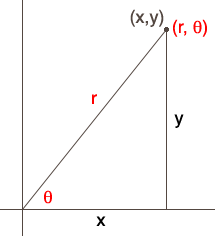SEARCH HOMEMath Central Quandaries & QueriesQuestion from Lan, a student: Given the rectangular equation (x^2)+2x+(y^2)+y=0, find the polar equation.Hi Lan,

The Cartesian coordinate system and the polar coordinate system are two ways to label points in the plane. If the origin of the Cartesian system is the same point as the pole of the polar system and the positive x-axis is the polar line then there is a nice relationship between the Cartesian coordinates (x, y) and the polar coordinates of the same point (r, θ) which you can read off the diagram.Pythagoras theorem tells us that x2 + y2 = r2. Also from the diagram you can see that x/r = cos(θ) and y/r = sin(θ). Hence the transformations you need are

x2 + y2 = r2, x = r cos(θ) and y = r sin(θ).

I drew the diagram with the point in the first quadrant and you should verify that these relationships are also valid in the other three quadrants. I think about polar coordinates with the angle measured in radians, counterclockwise from the polar line but you should check in your textbook to see what conventions are used there.

I hope this helps,
HarleyMath Central is supported by the University of Regina and The Pacific Institute for the Mathematical Sciences.